# The Mathematician

Sunday, October 3rd 2021, 5:11:58 pm

How Not to Be Wrong: The Power of Mathematical Thinking with Jordan Ellenberg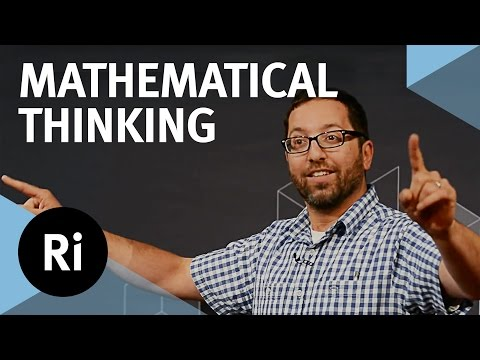Math is the hidden secret to understanding the world by Roger Antonsen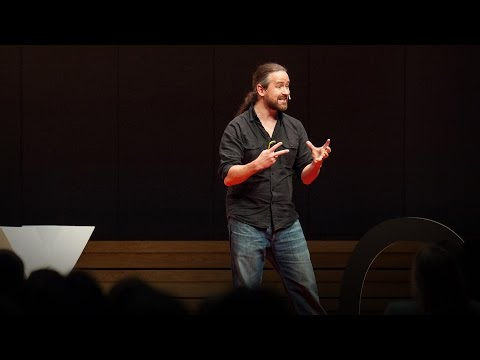50 Centuries in 50 minutes (A Brief History of Mathematics)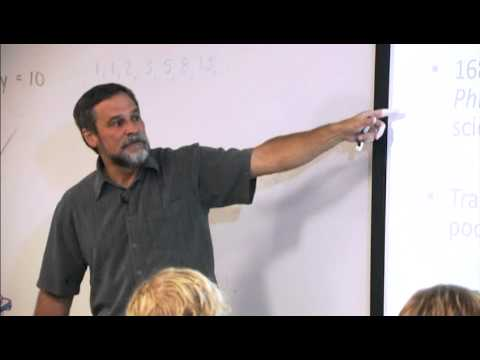The History of Mathematics and Its Applications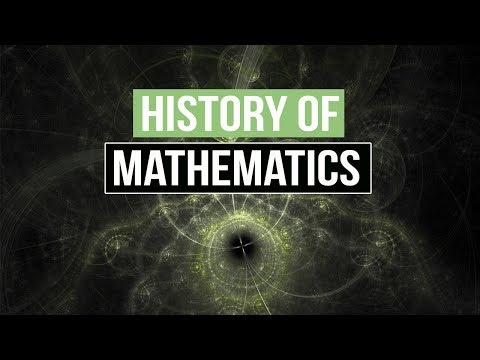How Karatsuba's algorithm gave us new ways to multiply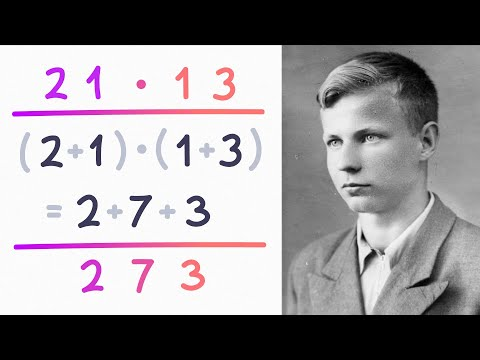The Meaning of Ramanujan and His Lost Notebook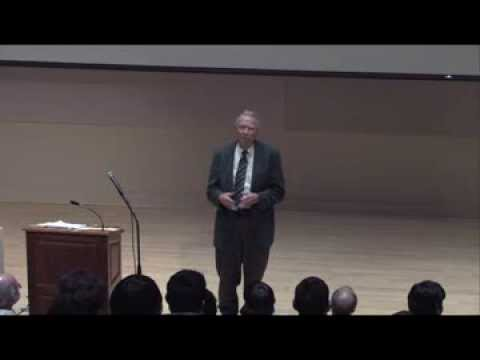Neil Turok Public Lecture: The Astonishing Simplicity of Everything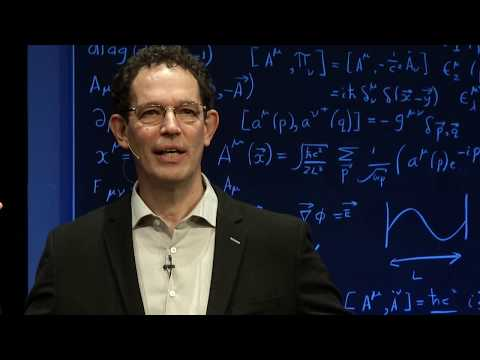Is math discovered or invented? - Jeff Dekofsky1. General Overview and the Development of Numbers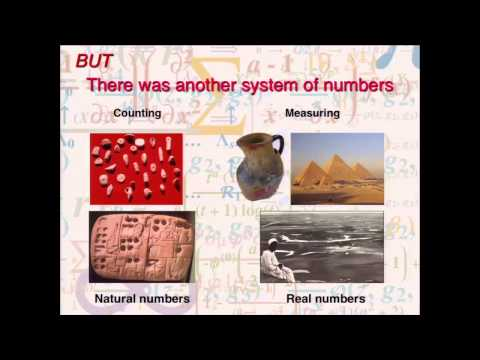What's the point of Geometry? - Euclid explains it nice and easy!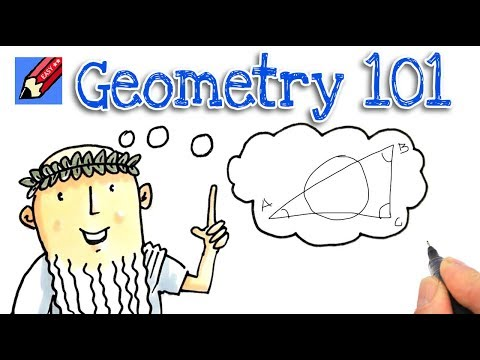Pythagoras Proves Himself (Explanation of what a Proof is by means of a visual proof)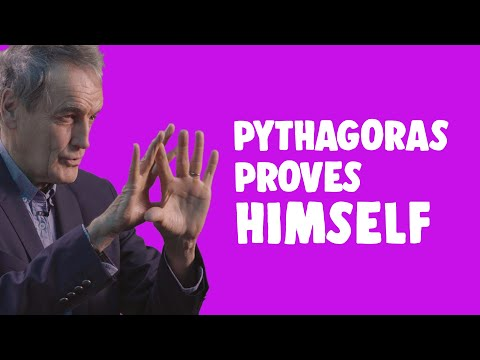Maths Made Easy! Pythagoras theorem: Basics [O&U Learn]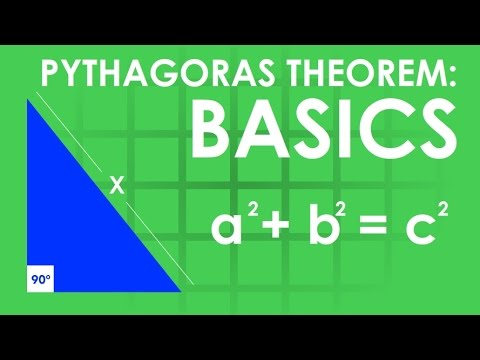Pythagoras' theorem (a) | Math History | NJ Wildberger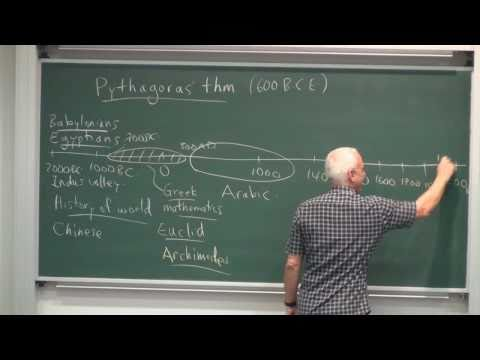The Golden Ratio (why it is so irrational) - Numberphile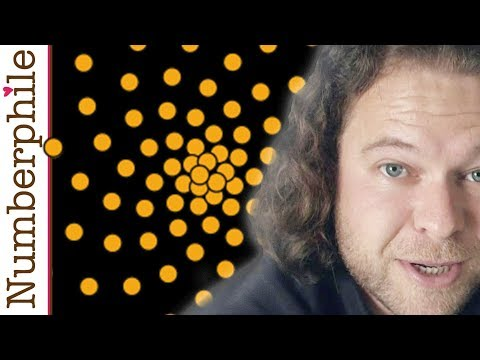The Golden Ratio & Fibonacci Numbers: Fact versus Fiction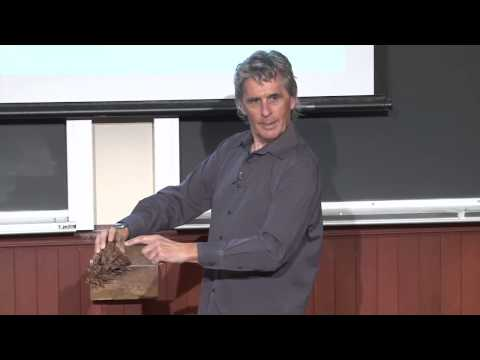The Origin of Graphs (Visualization of the fusion of Algebra and Geometry by means of Graphs)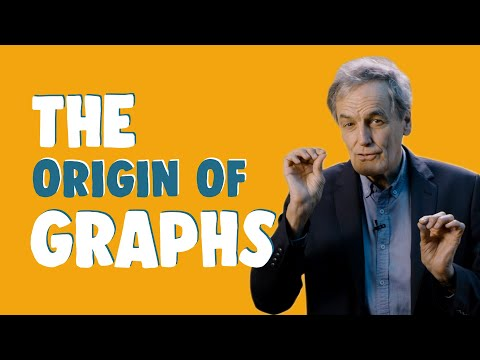Real Numbers And Their Graphs (Algebra 1 Tutor)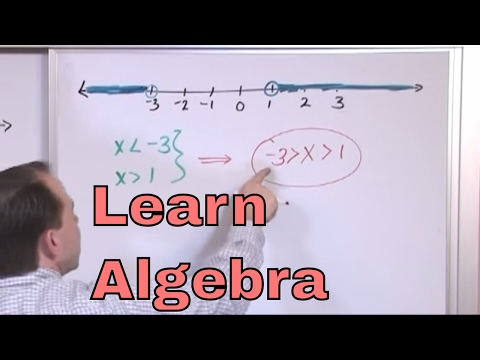Algebra Basics: Graphing On The Coordinate Plane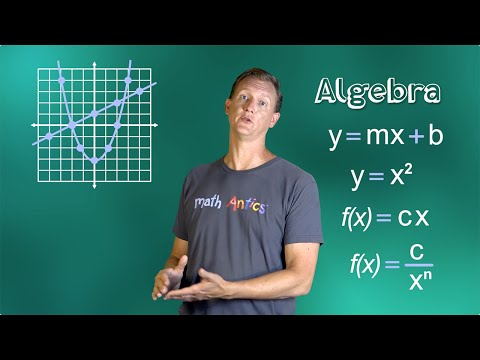Non-Euclidean Geometry Explained - Hyperbolica Devlog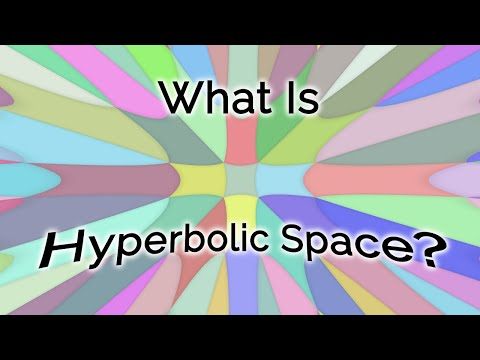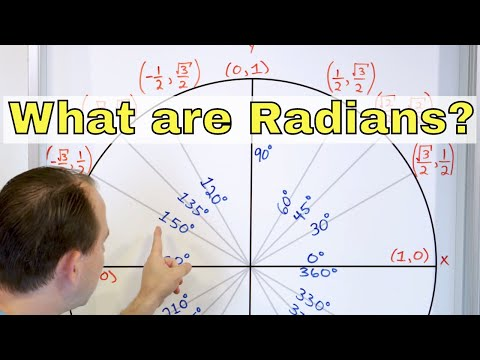Unit Circle - Definition & Meaning - Sin(x), Cos(x), Tan(x), - Sine, Cosine & Tangent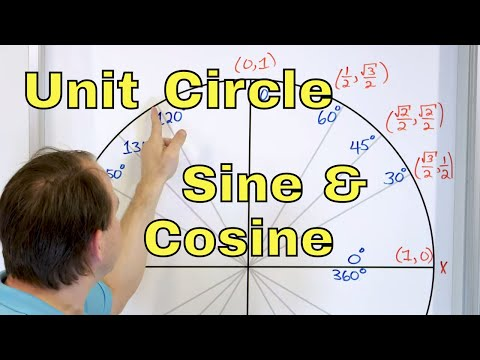Trigonometry Concepts - Don't Memorize! Visualize!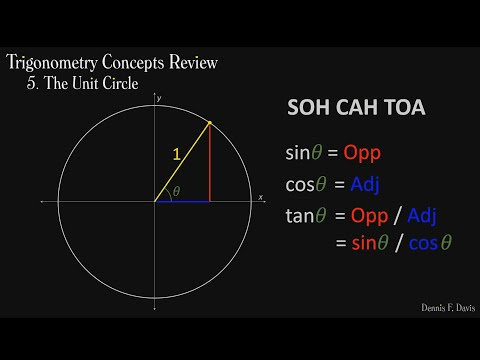The paradox at the heart of mathematics: Gödel's Incompleteness Theorem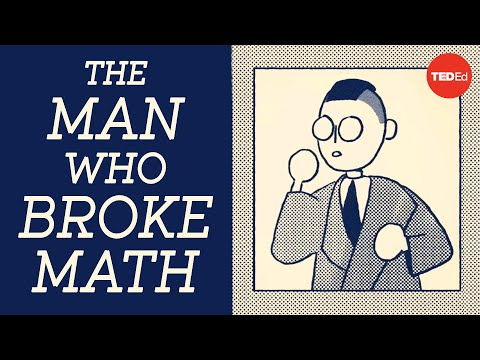Aristotle's Wheel Paradox - To Infinity and Beyond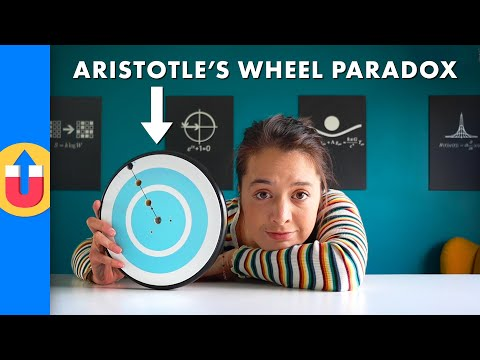Russell's Paradox - A Ripple in the Foundations of Mathematics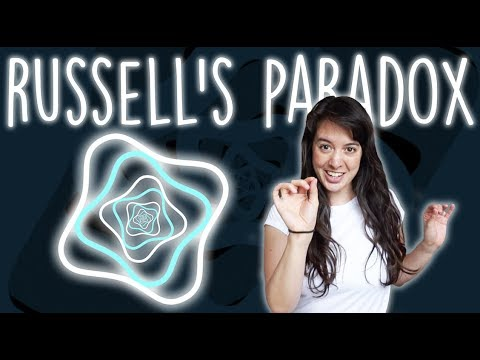Calculus: One of the Most Successful Technologies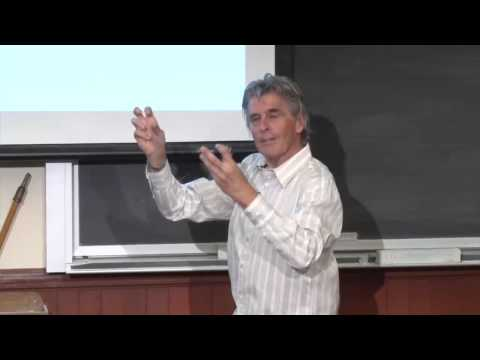Math Encounters -- On the Shoulders of Giants: Newton Revealed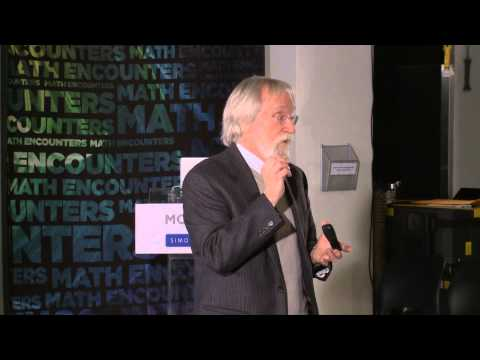Probability Theory - The Math of Intelligence #6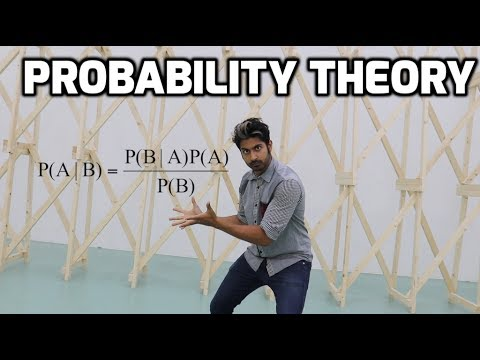How Science is Taking the Luck out of Gambling - with Adam Kucharski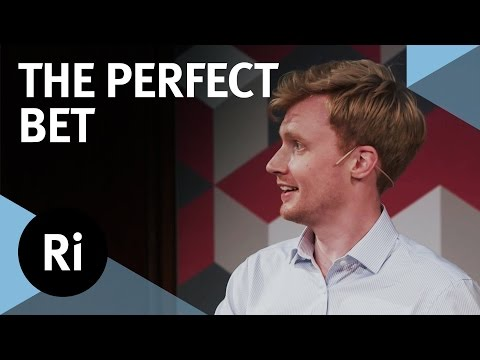Win a SMALL fortune with counting cards-the math of blackjack & Co.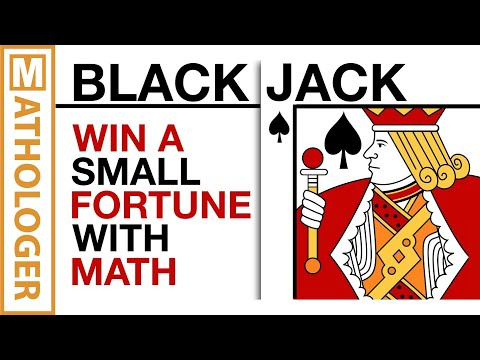How Galileo Unlocked The Doors to the Universe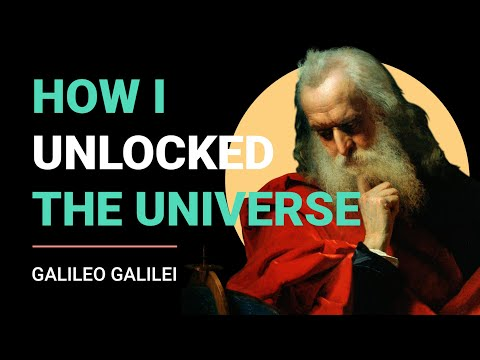The mathematical secrets of Pascal’s triangle - Wajdi Mohamed Ratemi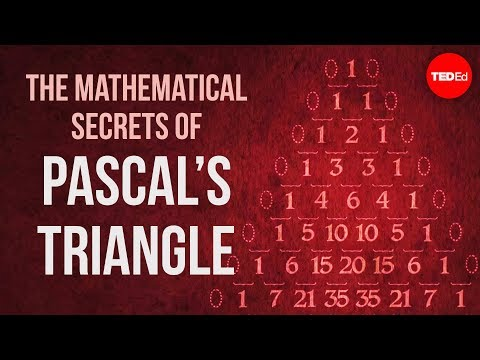21 - Pascals Triangle & Binomial Expansion - Part 1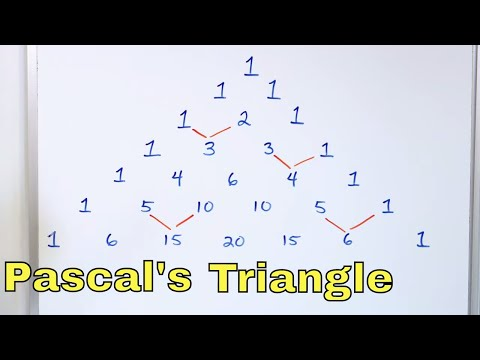Boolean Logic & Logic Gates: Crash Course Computer Science #3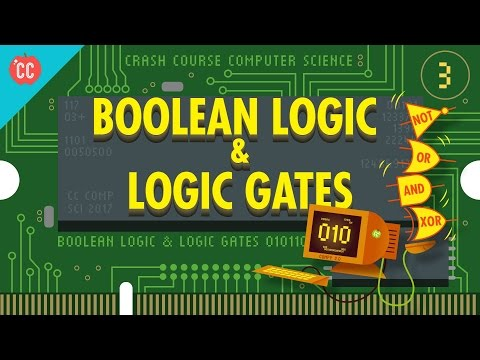History and Evolution of Cryptography and Cryptanalysis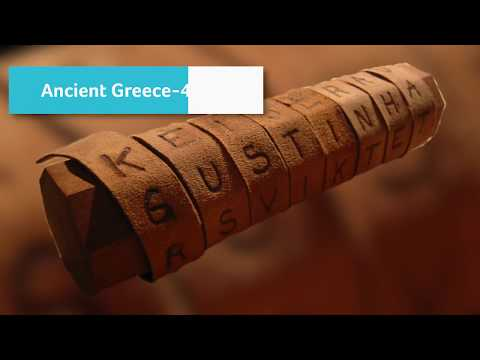Secret Codes: A History of Cryptography (Part 1)More Secret Codes: A History of Cryptography (Part 2)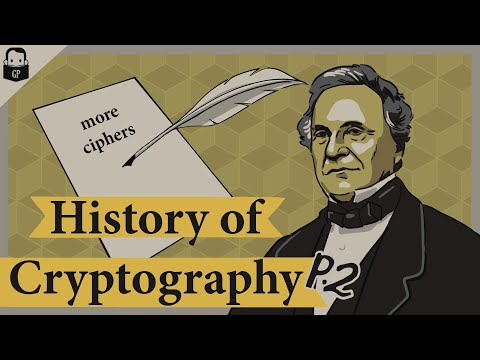JavaScript Algorithms Crash Course - Learn Algorithms & Big O from the Ground Up!Masterclass in JavaScript Algorithms and Data Structures (Part1)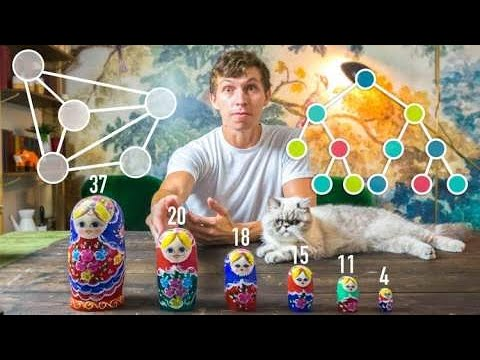Masterclass in JavaScript Algorithms and Data Structures (Part2)Algebra, Geometry, and Topology: What's The Difference?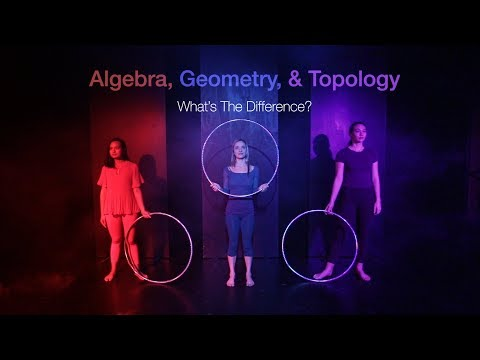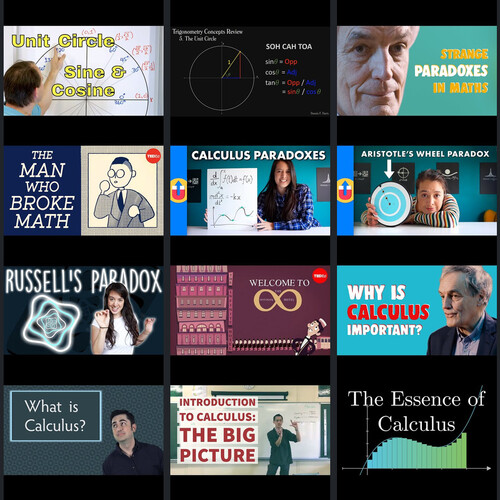#149: The Mathematician

Sunday, October 3rd 2021, 5:11:58 pm